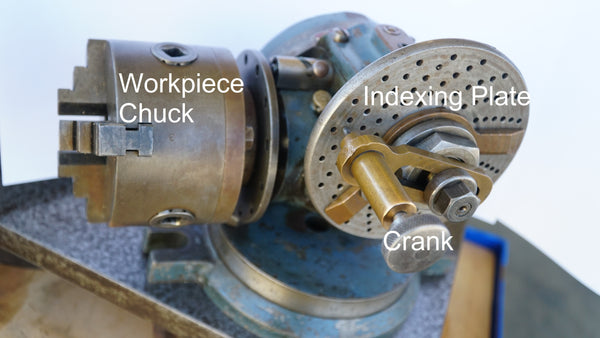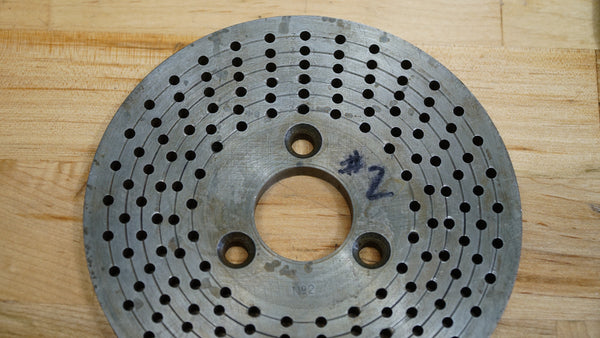# How to Use a Dividing (Indexing) Head

## What is a Dividing or Indexing Head?

Dividing Heads (often called indexing heads) are machine tools used to provide controlled and repeatable rotation to a tool or workpiece, usually as an accessory to a milling machine, grinder, or lathe. A classic and personal favorite of in our machine shop is the Ellis Dividing Head, but you can also find a different manufacturers dividing head that would fit your needs.  What makes it work inside the housing is a worm and worm gear transmission.  Regardless of the brand they usually come in gear ratios of 40:1 or 90:1.

Download the Evolvent Universal Dividing Head Chart 40:1 and 90:1 (PDF)Ellis Dividing Head - annotated original image

Dividing heads are closely related to rotary indexers, but with a key operational difference: Rotary indexers use a scale and show the angle of rotation (from 0 to 360 degrees), while dividing heads use pre-defined plates to rotate a fixed amount. These plates are generally circular with multiple rows of equally-spaced holes.

Using a dividing or indexing head correctly requires you to know the number of rotations depending on the project you're working on - you'd need to calculate how many full rotations, and how many holes in which circular pattern to go just the rotation you need. Fortunately, all of this math can be avoided by following the chart for your device.Plate #2 from an Ellis Dividing Head. Own work.

This plate can be used either directly, or through a geared dividing mechanism. In direct indexing the workpiece and plate rotate in a 1-to-1 ratio, and holes are used directly. That is, a plate with 12 holes can divide the workpiece into 2, 3, 4, 6, or 12 equal segments. A dividing head incorporates an internal gear ratio (usually 40:1, 60:1, or 90:1) with the same plates. In doing so, the dividing head enables many more combinations than just direct indexing.

For example, imagine a plate with 15 equally-spaced holes and a dividing head with a 40:1 gear reduction. In direct indexing, a workpiece could be divided into 3, 5, or 15 equal segments. Using the dividing head, the same workpiece could be divided into 2, 3, 4, 5, 6, 8, 10, 12, 15, 20, 25, 30, 40, 50, 60, 75, 100, 120, 150, 200, 300, or 600 segments. Essentially, the dividing head acts as if it’s a direct indexer with 600 holes; 15 holes in the actual plate * 40:1 gear ratio. Let’s look at how some of these combinations are possible.

With a 40:1 gear ratio, you’d need to turn the crank on the dividing head 40 full turns to rotate the workpiece once. To get 6 equal divisions, you’d need 40/6 = 6+2/3 = 6.66666 turns. The 6 turns are easy, but the remaining 0.66666 needs the holes on the plate. If we multiply the partial turn by the number of holes on the plate, we’ll know exactly how far to advance the diving head: 0.66666*15 = 10 holes. Putting these together, to divide the piece into 6 segments you would rotate the dividing head 6 turns and 10 holes.

To get 75 equal segments we’ll follow the same process: 40/75 = 0.53333 turns. Now, there is no full rotation, but 0.53333*15 = 8 holes. So if we rotate the dividing head forward by 8 holes each time, it will divide the workpiece into 75 equal segments.

In summary:

• Rotary indexer – uses continuous rotation and shows the angle at any position. Can be used to measure any angle, but requires careful and accurate measurement at each and every division
• Direct indexing – fast rotation and simple division, but limited range of divisions per plate
• Dividing head – more complicated division, but greatest number of options per plate

Download the Evolvent Dividing Head Chart 40:1 and 90:1 (PDF)